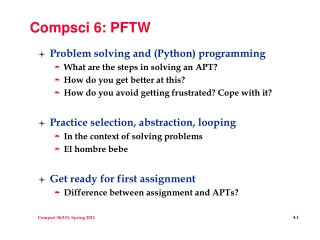DownloadDownload PresentationCompsci 6: PFTW

# Compsci 6: PFTW

Télécharger la présentation## Compsci 6: PFTW

- - - - - - - - - - - - - - - - - - - - - - - - - - - E N D - - - - - - - - - - - - - - - - - - - - - - - - - - -
##### Presentation Transcript

1. Compsci 6: PFTW • Problem solving and (Python) programming • What are the steps in solving an APT? • How do you get better at this? • How do you avoid getting frustrated? Cope with it? • Practice selection, abstraction, looping • In the context of solving problems • El hombre bebe • Get ready for first assignment • Difference between assignment and APTs?

2. How to solve an APT • Two very, very, very important steps • How to solve the problem with Paper, Pencil, (Calculator) • How to translate problem-solving to Python • Both steps can be hard, vocabulary and language are initially a real barrier • The more experience you have with Python, the easier step 2 will get • The more you understand the idioms and power of the language the more you can let step 2 influence step 1 • Step 1 is key, without it you won’t get anywhere

3. APT Pancake • How do you solve this problem? • First steps: are there simple cases that can be solved immediately? • What are these for the pancake problem? • How will you identify with Python? • Sometimes it helps to know if you are on track, use Python to check your paper and pencil work • Get specific, solve for 7, not N • Fix one parameter, vary the other • Identify the cases and continue

4. Three pancakes in a two-cake pan… • Number of cakes in the system • First 5 minutes • Number of cakes in the system • Second 5 minutes

5. Three pancakes in a two-cake pan… • Number of cakes in the system • Third 5 minutes • How many minutes to cook all three pancakes?

6. How to teach pancake flipping • http://www.youtube.com/watch?v=W_gxLKSsSIE • Is this computer science? • For longer, more complex robotic tasks • http://www.youtube.com/watch?v=4usoE981e7I • Back to specifics: • Capacity = 7 • Numcakes = 1,2,…7? • Numcakes = 8,9,10,11,12,13,14? • Numcakes = 15,16,17,18,19,20? • Is seven special? 6? 5? 3?

7. Eclipse Interlude • Finishing the Pancake problem • Translating problem-solving ideas to code

8. Lessons: special cases, abstractions • There are special cases in many, many problems • Identifying them is important • Abstracting them away when possible is important • Example: SilverDistance APT • Instead of four quadrants/cases, reducible to two? • Instead of (x,y) and (z,w) translate to (0,0) and (z-x,w-y) • Translating ideas into (Python) code • How do we create interesting “heads”, “totem poles” ? • How do create software for identikit? • How do we create Facebook, Foursquare, …

9. How do you solve a problem like …? • Translating English to Piglatin • Why is this fascinating? • http://www.google.com/webhp?hl=xx-piglatin • Is this like translating English to German? • Is it like translating Python to bytecode? • “downplay their unique quiet strength” • “ownplayday eirthay uniqueway ietquay engthstray” • What are the rules for pig-latin? See APT

10. APT Piglatin • How do you solve this problem? • First steps: are there simple cases that can be solved immediately? • What are these for the piglatin problem? • How will you identify with Python? • Words that begin with … • Vowel • Foods that begin with the letter ‘q’ for 200 Alex • Translation to Python • First ‘q’, then vowels

11. Three versions of is_vowel defis_vowel(ch): if ch =='e': return True if ch == 'a': return True if ch == 'i': return True if ch == 'o': return True if ch == 'u': return True return False def is_vowel(ch): c = "aeiou".count(ch) if c > 0: return True else return False def is_vowel(ch): return "aeiou".count(ch) > 0

12. Piglatin, age-stay one-way def convert(s): if s == 'q': return s[2:]+"quay" if is_vowel(s): return s+"way" • Review from last lab: slicing, concatenation, index • Where does string-indexing start? • What does slice with a single parameter do?

13. Piglatin, age-stay o-tway def convert(s): if s == 'q': return s[2:]+"quay" if is_vowel(s): return s+"way" if is_vowel(s): return s[1:]+s+"ay" if is_vowel(s): return s[2:]+s[:2]+"ay" if is_vowel(s): return s[3:]+s[:3]+"ay" if is_vowel(s): return s[4:]+s[:4]+"ay"

14. Piglatin, age-stay ee-threay def convert(s): if s == 'q': return s[2:]+"quay" if isvowel(s): return s + "way" for index in range(1,len(s)): if isvowel(s[index]): return s[index:]+s[:index]+"ay" • Generalize/parameterize by what varies • What does a loop do? it repeats!

15. What does this do? defchangeup(s): rep = "" for ch in s: rep = rep + ch*2 return rep • What's new here, what's similar to what we know? • What does it mean to loop over a string? • How do we know it's a string? • Code experiments to help reason (or just type and run?) • Look again at Uppity.py?

16. Dawson Engler • ACM Hopper Award 2008 "In his papers on automated program checking, Dawson Engler introduces and develops powerful techniques and tools for practical program analysis for finding errors in code." • Started coverity.com • Very successful startup to find errors in code • http://myvideos.stanford.edu/player/slplayer.aspx?course=CS240&p=true